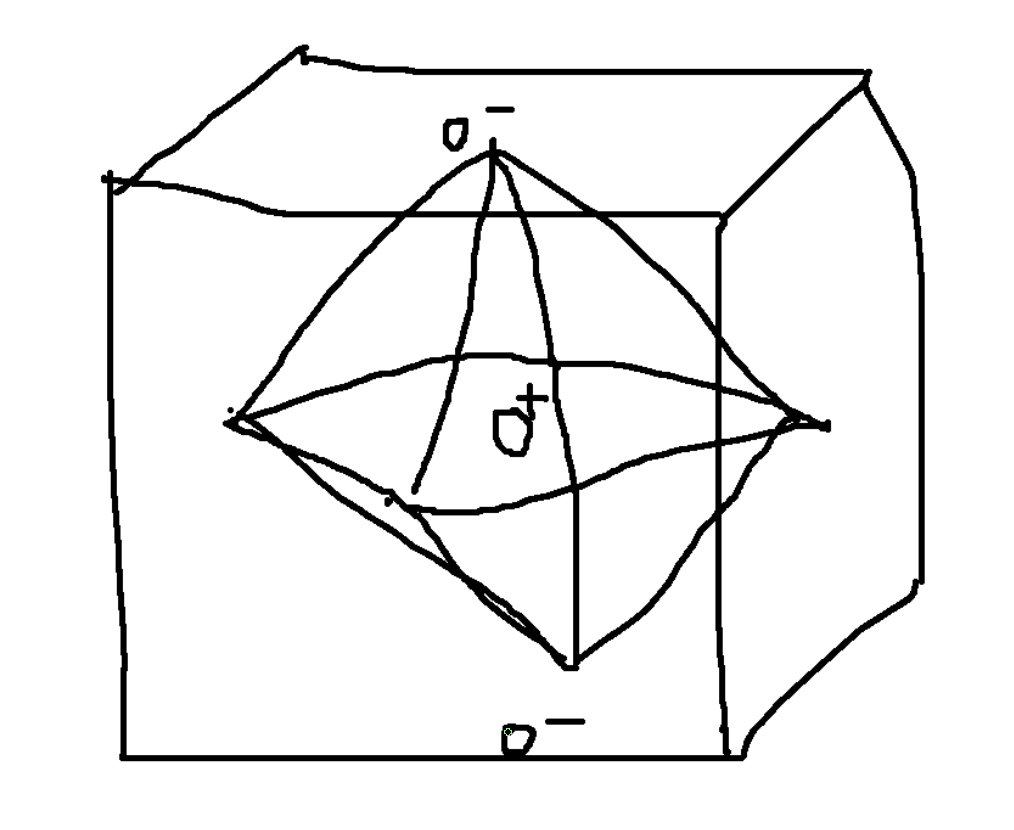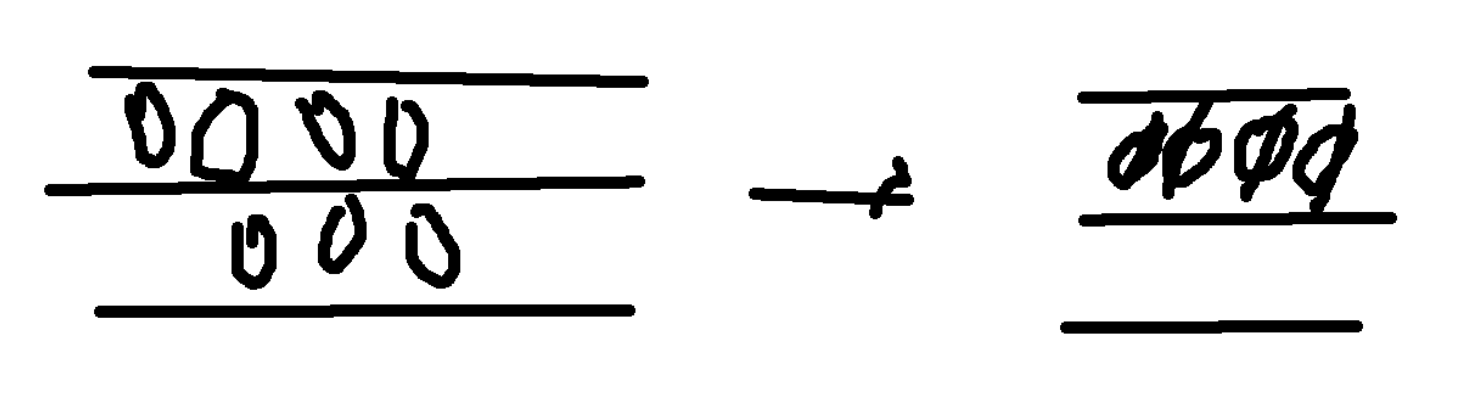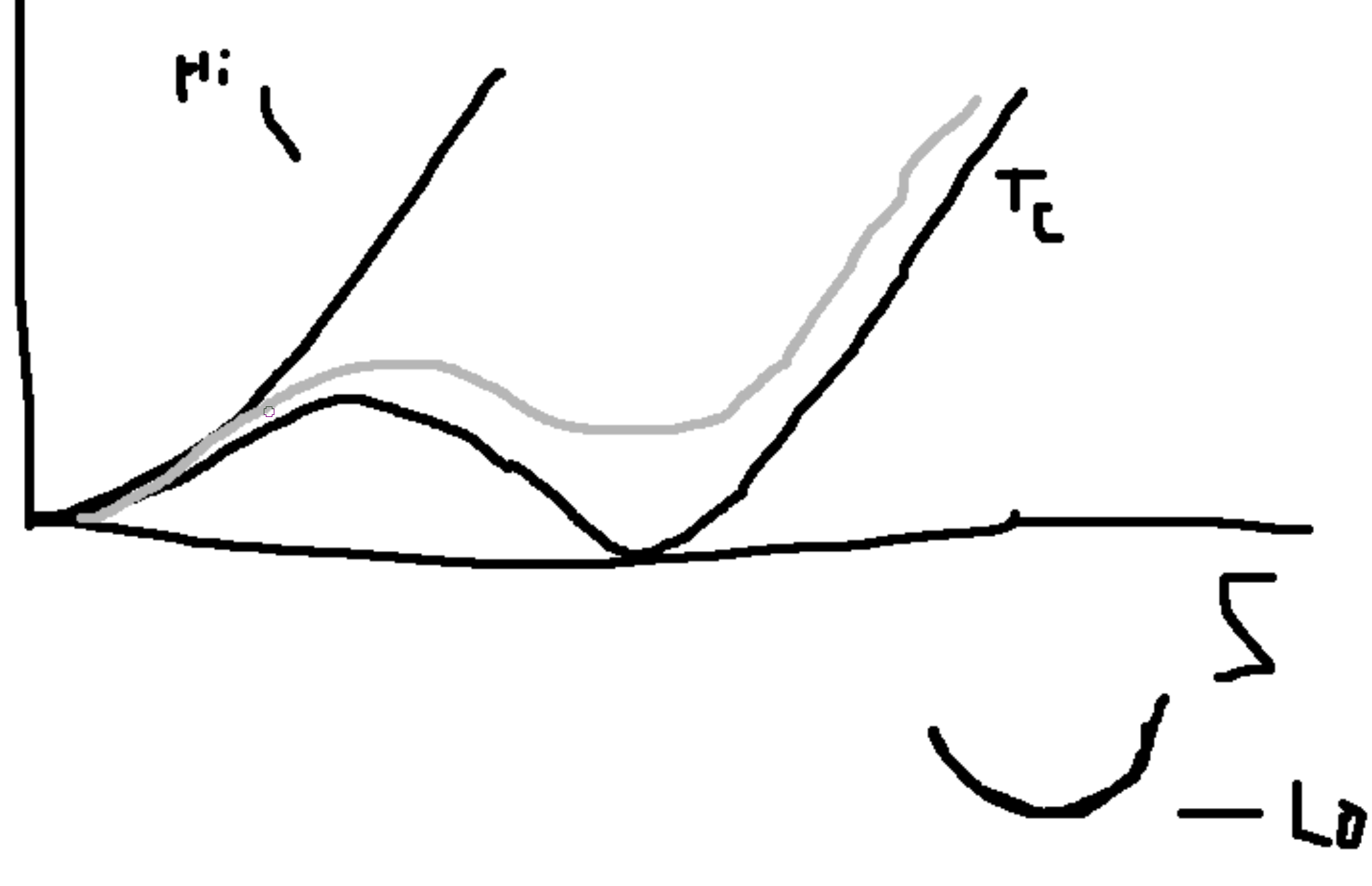# Symmetries and Order Parameters

Start out with a system (with properties and dynamics) described by its Hamiltonian $$\mathcal{H}$$. $$\mathcal{H}$$ is invariant under some group $$G$$, which give the symmetries of $$\mathcal{H}$$.

A system can have states with symmetries lower than the full group of the Hamiltonian, i.e. the state is invariant under some subgroup $$G$$. We call those symmetry breaking.

Our state is described by thermodynamic (ensemble) averages of operators $$\phi_\alpha$$, the ensemble average is then $$\langle\phi_\alpha\rangle,\langle\phi(x)\rangle$$ which is not invariant under the full group $$G$$.

These averages (⟨Φ⟩) are called order parameters. $$\langle\phi\rangle$$ is a global order parameter and $$\langle\phi(x)\rangle$$ is a local order parameter.

We can choose that the global order parameter is zero for high symmetry (always the full group, hence high T).

### Symmetries

Discrete symmetries $$Z_2$$, group 2 integers. Ex. Ising symmetries, uniaxial (anti-)ferromagnetic system. FM: $$(\langle m_z\rangle)$$. AFM: $$\langle m_{z,A}-m_{z,B}\rangle$$. Ex: Rb $$_2$$ NiF $$_4$$, K $$_2$$ MnF $$_4$$. Order-disorder: Binary-order $$\langle n_A-n_B\rangle$$ $$[\beta-\text{brass}]$$. Liquid-gas: $$\langle n_L-n_g\rangle$$.

Dispacive: (Ferroelectric) perouskite $$\langle n_z\rangle$$ BaLiO$$_3$$.$$Z_3$$ group 3 integers. Graphene/ graphite surface (Krypton occupations on graphene lattice).$$Z_N$$ group $$N$$ integers.

Continuous Symmetries:

• Rotations on a plane: O $$_2/U(1)\to$$ Phase of a complex number. Examples: Easy plane FM, Easy plane AFM, Bose-Einstein condensation (superfluid), various liquid crystal phases.$$O_3$$ rotations in 3D, Heisenberg FM.

### Axioms of Group

Set $$G$$ with binary operatorion $$\cdot$$. The group is $$(G,\cdot)$$.

• $$a(bc) = a(bc)$$
• $$a\cdot i = a$$
• $$a\cdot a^{-1} = i$$

Subgroup: Subset $$H$$ to $$G$$, $$H$$ is a subscrube of $$G$$ if $$(H,\cdot)$$ is also a group.

## Towards Landau/ Landau-Gurburg Theory

$$F(\phi(x)) = \int d\overline{x} f(n(\overline{x}))$$

Consider if we have an ideal gas. Subdivide the space into boxes of size $$\Delta V$$. Then for $$n_j$$ points in the box at position $$\vec{x}_j$$ we have the density, $$\rho(\vec{x}_j) = \frac{n_j}{\Delta V}$$.

Consider a spatially inhomogenous system. (Ideal gas) with free energy $$F(T,V,N) = NkT\left(\ln\left(n\lambda^3\right)-1\right)$$. $$N_j = n(\vec{x}_j)\Delta(V)$$. $$f^{ig}=\frac{F(T,V,N)}{\Delta V} = n(\vec{x}_j)kT\left(\ln\left(n(\vec{x}_j)\lambda^3\right)-1\right)$$ $$n(x), F[n] = \int f(n(x))dx$$. We integrated out microscopic degrees of freedom and replaced with coarse-grained field. Can calculate any equilibrium property that can be written in terms of $$n(x)$$. So, what is $$p(n(x)) = ?$$. From before, $$\alpha\to p_\alpha(H)$$. $$n(x)\to p(n(x))$$ which is governed by the free energy $$F$$ of the densities. $$p(n(x)) = \frac{1}{Z}\exp(-\beta F[n(x)])$$. Driving force (to equilibrium)? $$\mu = \frac{\partial F}{\partial N} = \frac{\partial F/V}{\partial N/V} = \frac{\partial f}{\partial n}$$. Then $$\mu(x) = \frac{\delta F[n(y)]}{\delta n(x)} = \frac{\delta}{\delta n(x)} \int f(n(y))dy$$, which is called the variational/ functional derivative. Then, $$\mu(x) = kT\ln (n\lambda^3)$$.

Considering currents. $$j = n\cdot v$$, $$v \propto \frac{\partial\mu}{\partial x}$$, $$v = -\gamma\frac{\partial\mu}{\partial x}$$. $$\gamma$$ relates to mobility and the negative relates to downhill. So, $$v = -\gamma n(x) \frac{\partial kT\ln(n(x)\lambda^3)}{\partial x} = -\gamma n(x)kT\frac{1}{n}\frac{\partial n}{\partial x}$$. From the continuituy equation, $$\nabla\cdot J + \frac{\partial n}{\partial t} = 0$$. Putting this together we get $$\frac{\partial n}{\partial t} = \gamma kT\frac{\partial^2 n}{\partial x^2}$$.

$$F = \int dx f(T,\langle\phi(\overline{x})) + \int dx \frac{1}{2}k(\nabla\langle\phi(x)\rangle^2)$$. Landau’s theory: ignore the second term. Including the second term is L-G.

So expanding, $$f(T,\phi) = f_0(T) + f_1\phi + f_2\phi^2 + f_3\phi^3 + f_4\phi^4 + \cdots$$ where the coefficients $$f$$ can depend on temperature, except:

1. Highest power of $$\phi$$ provides a bounding potential: $$\phi^{2n}, f_{2n}>0$$. So that the order parameter remains finite.
2. $$f_1$$: example $$\phi = m = \frac{M}{V}$$. $$F(T,V,M) \Rightarrow dF = -SdT - pdV + BdM$$ $$\frac{\partial F}{\partial M} = \frac{\partial f}{\partial m} = B$$. For high temperature and no field, the magnetization should be zero, hence $$\phi=0$$. Then, $$\frac{\partial f}{\partial m}|_{m=0} = 0$$ since it is a free energy minimum. Therefore, $$f_1 = 0$$. Hence: $$f(T,\phi) = f_0(T) + f_2\phi^2 + f_3\phi^3 + f_4\phi^4 + \cdots$$. $$f(T,\phi)\equiv$$ Landau free energy density. This is typically written: $$f = f_0(T) + \frac{1}{2}\mu(T)\phi^2 + \frac{1}{3!}f_3\phi^3 + \frac{1}{4!}g\phi^4$$ by theorists and partical physics. $$f = f_0(T) + \frac{1}{2}r\phi^2 - w\phi^3 + u\phi^4$$. $$f = f_0(T) + \frac{1}{2}b(T)\phi^2 + \frac{1}{3}c(T)\phi^3 + \frac{1}{4}d\phi^4$$.

Aside: MFT gives qualitative pictures that are pretty accurate to expectation.

## Landau Model for Neumatic to Isotropic

Phase transition in liquid crystals.

Isotropic: any orientation of liquid crytals. Neumatic: molecules predominantly align with some axis $$\hat{n}$$.

### Tensor Model - Neumatic to Isotropic Phase Transition in Liquid Crystal

Let $$\hat{n}$$ be a global unit vector called the Frank director. Let $$\hat{v}^\alpha$$ be the unit director for the $$\alpha$$ molecule’s orientation.

Ordering is a measure of how close $$\hat{v}^\alpha$$ is to $$\hat{n}$$, $$\hat{v}^\alpha\cdot\hat{n} = \cos\theta$$. For perfect order, $$\theta=0,\pi$$ so $$\cos^k\theta$$ with $$k$$ even.

Want $$f = f_0 + f_1\phi + f_2\phi^2 + f_3\phi^3 + f_4\phi^4$$.

In the high-temperature phase, $$f_1=0$$.

Let $$\mathcal{Q}$$ be a tensor. Then, $$\phi^n = \text{Tr}\{\mathcal{Q}^n\}$$. In high T phase $$\to \phi (high) = 0$$. So, $$\text{Tr}\{\mathcal{Q}\} = 0$$ for high temperature. So our outer product, $$\vec{v}\cdot\vec{v}^T$$. Start with, $$\mathcal{Q}_{ij} = \sum_\alpha v_i^\alpha v_j^\alpha$$. So, $$\mathcal{Q}(\vec{s}) = \frac{1}{\frac{N}{V}}\sum_\alpha v_i^\alpha v_j^\alpha\delta(x-\overline{x}^\alpha)$$. Note the tensor must be symmetric.

Absolute disorder: $$\theta$$ is uniformly distributed, $$\int d\Omega S(\theta) = 0$$.

Absolute order, $$\forall\alpha.\vec{v}^\alpha\|\hat{n}$$.

Choose a coordinate system such that the first basis vector is $$\hat{n}$$. So perfect alignment is $$\vec{v}^\alpha = (1\: 0\: 0)$$.

In order state, $$v^{\alpha T}v^\alpha\text{Tr}\left(\begin{pmatrix} 1 & 0 & 0 \\ 0 & 0 & 0 \\ 0 & 0 & 0 \end{pmatrix} + \begin{pmatrix} -1/3 & 0 & 0 \\ 0 & -1/3 & 0 \\ 0 & 0 & -1/3 \end{pmatrix}\right) = 0$$.

$$\text{Tr}\left(\begin{pmatrix} S & 0 & 0 \\ 0 & 0 & 0 \\ 0 & 0 & 0 \end{pmatrix} + \begin{pmatrix} -S/3 & 0 & 0 \\ 0 & -S/3 & 0 \\ 0 & 0 & -S/3 \end{pmatrix}\right) = \text{Tr} \begin{pmatrix} 2/3S & 0 & 0 \\ 0 & -1/3S & 0 \\ 0 & 0 & -1/3S \end{pmatrix}$$.

Then, $$\langle\mathcal{Q}_{ij}\rangle = S\left(n_in_j - \frac{1}{3}\delta_{ij}\right)$$

$$\int d\Omega S(\cos^k\theta) = 0 = \int d\varphi\int d\theta\sin\theta S(\cos^k\theta) = 2\pi\int d\theta\sin\theta S(\cos^k\theta)$$ with $$S(\cos^k\theta) = 1$$ for $$\theta=0,\pi$$. $$S = \frac{1}{2}\langle(3(v^\alpha\cdot\hat{n})^z-1)\rangle = \frac{1}{2}\langle (3\cos^2\theta^\alpha-1)\rangle$$.

Trace: $$\text{Tr}\mathcal{Q}^2 = \frac{4}{9}S^2 + \frac{1}{9}S^2 + \frac{1}{9}S^2 = \frac{2}{3}S^2$$. Trace: $$\text{Tr}\mathcal{Q}^3 = \frac{8}{27}S^3 - \frac{1}{27}S^3 - \frac{1}{27}S^3 = \frac{6}{27}S^3 = \frac{2}{9}S^3$$. Trace: $$\text{Tr}\mathcal{Q}^4 = \frac{16}{81}S^4 + \frac{1}{81}S^4 + \frac{1}{81}S^4 = \frac{18}{81}S^4 = \frac{2}{9}S^4$$.

$$f(S) = \frac{1}{2}rS^2 - wS^3 + US^4$$.

$$\frac{1}{2}r\frac{3}{2}\text{Tr}\mathcal{Q}^2 - w\frac{9}{2}\left(\text{Tr}(\mathcal{Q}^3) + U\frac{9}{2}\text{Tr}(\mathcal{Q}^4)\right)$$.

$$S = \frac{1}{2}\langle(3\cos^2\theta^\alpha-1)\rangle$$$$L = \int dQ$$, $$dQ = TdS$$.

## Ising Model Aside

$$m^2\to m^4\to m^4m^2m^2m^2m^4$$.

$$f = f_0 \neq f_2(T)m^2 + f_4m^4$$ where $$f_4>0$$. $$f_2(T-T_C)$$. $$f_2>0$$ for $$T>T_C$$ and $$f_2<0$$ for $$T Can approximate \(f_2$$ for $$T\to T_C$$ by a linear approximation, $$f_2\approx a(T-T_C)$$.

## Functional Aside

C.f. Entropy, $$S = -k\sum_i p_i\ln p_i = -k\int dx p(x)\ln p(x)$$, $$\frac{\partial S}{\partial p_i} = -k(\ln p_i + 1) = S[p(x)] = -k(\ln p(x) + 1)$$.

Functional derivatives, for functions $$f$$ that can be Taylor expanded about $$x$$, $$\frac{\delta F[f(x)]}{\delta f(y)} = \lim_{\epsilon\to 0} \frac{F[f(x) + \epsilon\delta(x-y)] - F[f(x)]}{\epsilon} = \lim_{\epsilon\to 0} \frac{\int f(x)+\epsilon\delta(x-y) dx - \int f(x)dx}{\epsilon} = \lim_{\epsilon\to 0} \frac{\int \epsilon\delta(x-y) dx}{\epsilon} = 1$$. In our case, $$F[n(x)] = \int f(n(x))dx$$. So, $$\frac{\delta F[n(x)]}{\delta n(y)} = \lim_{\epsilon\to 0}\frac{\int f(n(x)+\epsilon\delta(x-y))dx - \int f(n(x))dx}{\epsilon} = \lim_{\epsilon\to 0}\frac{\int \left(f(n(x)) + \frac{\partial f}{\partial n}(\epsilon\delta(x-y)) \mathcal{O}(\epsilon^2)\right)dx - \int f(n(x))dx}{\epsilon} = \lim_{\epsilon\to 0}\frac{\int \frac{\partial f}{\partial n}(\epsilon\delta(x-y))dx}{\epsilon} = \lim_{\epsilon\to 0}\int \frac{\partial f(n(x))}{\partial n(x)}(\delta(x-y))dx = \frac{\partial f(n(y))}{\partial n(y)}$$.

To Try: $$F[\phi(x)] = \exp\left(\int\phi(x)f(x)dx\right)$$, then what is the functional derivative $$\frac{\delta F[\phi(x)]}{\delta \phi(y)}=\cdots = f(y)F[\phi(x)]$$.

For one with a gradient: $$F[n] = \int f(\overline{x},n(\overline{x}),\nabla n(\overline{x}))dx$$, $$\frac{\delta F[n]}{\delta n(x)} = \frac{\partial f}{\partial n} - \nabla\cdot\frac{\partial f}{\partial\nabla n}$$.

Aside. For most field theories, we just postulate that $$p(\phi(x)) = \frac{\exp(-\beta \mathcal{H}_{IG}(\phi(x)))}{Z}$$ exists but we can show it for a few. $$Z = \int(\mathcal{D}\phi)\exp(-\beta\mathcal{H}(\phi))$$.

## Multicritical Points

$$f = \frac{1}{2}r\phi^2 + u_4\phi^4$$, $$u_4>0$$.

When $$r$$ changes sign you get a continuous phase transition.

Consider the model, $$f = \frac{1}{2}r\phi^2 + u_4\phi^4 + u_6\phi^6$$, $$u_6>0$$ and $$u_4$$ can be either sign. Plotting $$r$$ versus $$u_4$$,

Created: 2023-06-25 Sun 02:29

Validate